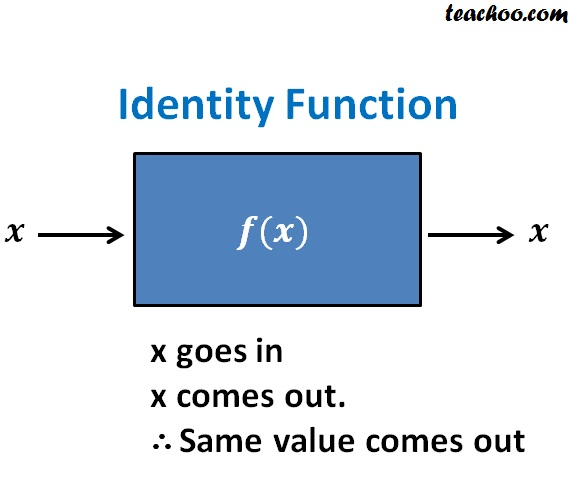Finding Inverse

Chapter 1 Class 12 Relation and Functions
Concept wise

Identity function is a function which gives the same value as inputted.Example

f: X → Y

f(x) = x

Is an identity function

We discuss more about graph of f(x) = x in this post

## Find identity function of fog and gof

f: X → Y

& g: Y → X

gof

gof = g(f(x))

gof : X → X

We input x

So, we should get x

gof = x

We write gof = I X

where I X is the identity function in set X

fog

fog = f(g(y))

fog : Y → Y

We input y

So, we should get y

∴ fog = y

We write fog = I Y

where I Y is the identity function in set Y

## Find Identity Function of set X and set Y

X = {1, 2, 3}

Y = {4, 5, 6}

In identity function,

we get what we input

If we input 1, we should get 1

#### Identity function of set X

If we input 1, we should get 1

If we input 2, we should get 2

If we input 3, we should get 3

∴ I X = {(1, 1), (2, 2), (3, 3)}

Identity function of set Y

If we input 4, we should get 4

If we input 5, we should get 5

If we input 6, we should get 6

∴ I Y = {(4, 4), (5, 5), (6, 6)}

Learn in your speed, with individual attention - Teachoo Maths 1-on-1 Class